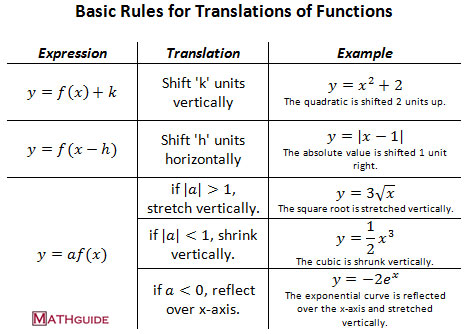Translations / Transformations
 Home > Lessons > Translations/Transformations Search | Updated September 16th, 2019
Introduction

In this lesson, you will learn how to determine the translational (transformational) attributes on the graphs of functions by examining the modifications on those functions. Here are the sections within this lesson:

 Basic Rules of Translations / Transformations Graphs of functions can be shifted horizontally and vertically, stretched and shrunk vertically, and reflected over the x-axis according to this table.Instructional Videos View our instructional video on translations (transformations).ideo: Basic Translations (Transformations) of Functions Interactive Quizzes Try this interactive quizzes to determine if you understand translations (transformations).uiz: Parabolic Translations: From Equations to Statementsuiz: Parabolic Translations: From Statements to Equationsuiz: Radical Functions: Translations, Domain, and Range Related Lessons Try these lessons, which are closely related to the sections above.esson: Translating Exponential Functionsesson: Relationsesson: Functionsesson: Testing Relations for Symmetryesson: Graphing Logarithmic Functionsesson: The Rational Root Theorem